August 9, 2022

# C Program to Find the Roots of a Quadratic Equation

Detailed question:
Design and develop a C program that accepts three coefficients (a, b, and c) of a Quadratic equation (ax2 +bx +c=0) as input and compute all possible roots and print the possible roots for a given set of coefficients. Also print the message in case of zero valued coefficient/s.

Roots of a quadratic equation are given by,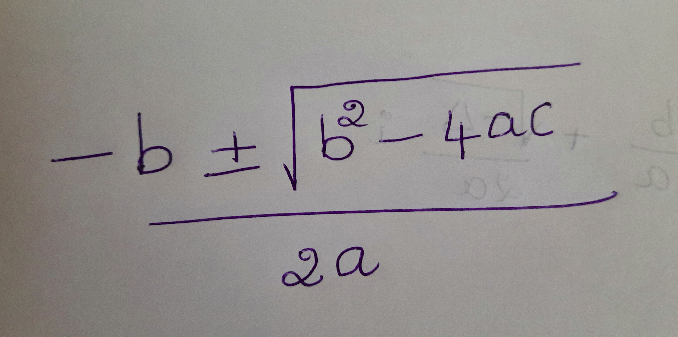Here b2-4ac is knows as the discriminant.
If the discriminant is greater than zero, then we will get two real roots.
If the discriminant is equal to zero, then we will get one real root.
If the discriminant is less than zero, then we will get 2 complex roots. example:
So, we will carefully examine the above equation to find the roots of a quadratic equation.
a should not be zero. Since we can not divide any number by zero. So, we have to validate, if the input value of a is zero or not.

Steps to follow:
1: Get input from user for the 3 coefficients :a , b, c
2: Exit application if the value of a is zero.
3: Find out the discriminant.
4: Use different logic to find the roots based on the value of discriminant.
5: Display the value.

Run below code.

```#include <stdio.h>
#include <math.h>

int main() {

float a,b,c;
printf("Enter 3 coefficients: a,b,c. Press enter after entering each numbers.\n");
scanf("%f %f %f", &amp;a,&amp;b,&amp;c);

if(a ==0){
printf("\nValue of 'a' should not be zero");
return 0;

}

//Finding the value of Discriminant.
float discriminant = b*b - (4*a*c);
if(discriminant >0){
printf("We will get two real solution \n");

float res1 = (-b +sqrt(discriminant))/(2*a);
float res2 = (-b - sqrt(discriminant))/(2*a);
printf("roots are\n");
printf("%f \n", res1);
printf("%f \n", res2);

}else if(discriminant <0){
printf("We will get pair of complex solution. \n");
float firstPart = -b/(2*a);
float val = sqrt(-discriminant);
float secPart = val/(2*a);

printf("%.2f + %.2f i\n", firstPart, secPart);
printf("%.2f - %.2f i\n", firstPart, secPart);
//printf("%.2f i\n", val);

}else{
printf("We will get just one real solution. \n");
float res1 = (-b)/(2*a);

printf("Root is\n");
printf("%f \n", res1);
}
return 0;
}

```

Let us check the application in detail.
Get the user input for 3 coefficients: a,b,c. Created a float variable a, b, c and store the user input to these variables respectively. (Line no: 6 to 8) You need to use float variable. Since real number can have decimal numbers too. example : 1.5, -6.88 etc.

If the value of a is zero, exit the application. (Line no: 10-12). Find the value of discriminant. Line no: 17
Based on value of discriminant, we have to use different logic. That is why I have used if condition.
Part 1: If the value of discriminant is more than zero, then we will get two real solution.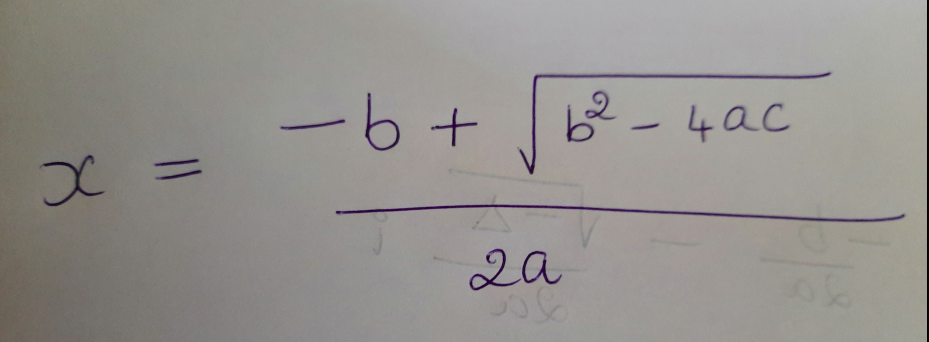sqrt is a function declared in the standard header: math.h
Line no: 21, 22. Use the formula to get the result and save it in float variable res1, res2. Display the values.

Part 2: If value of discriminant is less than zero, we will get complex solutions. example : -0.4 + 0.85i , -0.4 – 0.85i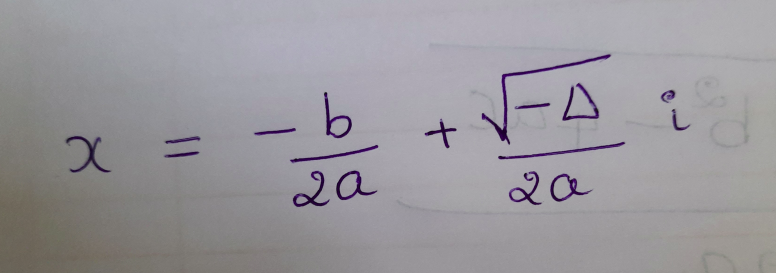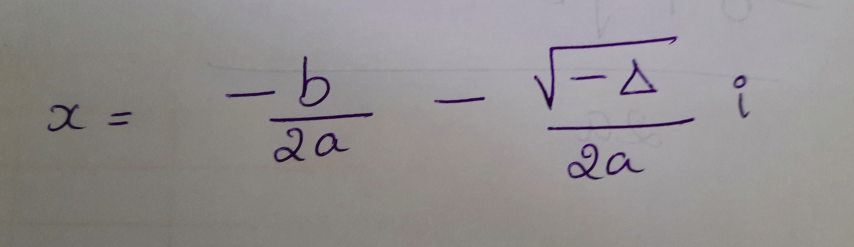How to get the result in this case? sqrt function will not accept negative number.
One more thing: This is pure mathematics.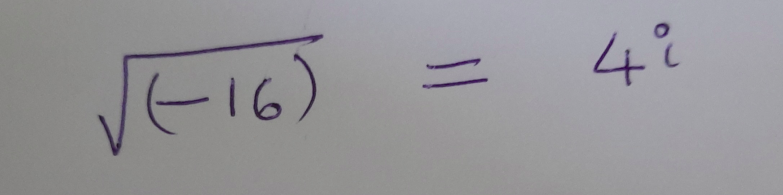So, if we get any negative number and if we need to find out the square root of negative number, we will find the square root of positive number, then add i to the result 🙂
Solution has 2 parts. First part is -b/2a Second part is sqrt(positive value of discriminant). Then display the result as firstPart + secondPart i , firstPart – secondPart i

Part 3: If the value of discriminant is zero, then we will get one real solution. result is -b/2a Display this value.

Compile the application using the command: gcc quadratic.c
Note: quadratic is the C file name. If you are running the application in Linux, then use the command: gcc quadratic.c -lm
Reason : Refer the link: https://stackoverflow.com/questions/10409032/why-am-i-getting-undefined-reference-to-sqrt-error-even-though-i-include-math
Run the application using: ./a.out (in Linux) >a in windows#### Mahesh

View all posts by Mahesh →